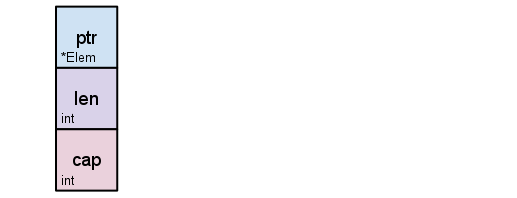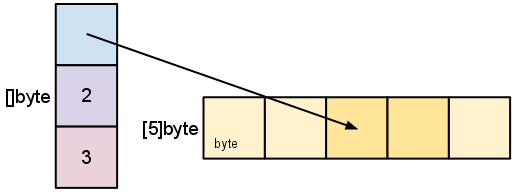golang中的rune

rune这个概念困扰我好久了。。。中文直译字面量给我的感觉就是犇猋骉麤

fmt.Println(1,"a",'a')
//output:1 a 97

小屋终于有了小绿锁！！！

openssl x509 -noout -modulus -in subdomain_domain_com.crt | openssl md5
openssl rsa -noout -modulus -in server.key | openssl md5

15块。。。估计够用挺久了吧 =.= 后面有时间要把所有都替换为https

PHP 中的单双引号

php 果然很有趣

<?php
$a1 = null;$a2 = 'null';
$a3 = "null";$a4 = 0;
$a5 = '0';$a6 = "0";
$a7 = array();$a8 = array(array());
echo empty($a1) ? 'true' : 'false'; echo empty($a2) ? 'true' : 'false';
echo empty($a3) ? 'true' : 'false'; echo empty($a4) ? 'true' : 'false';
echo empty($a5) ? 'true' : 'false'; echo empty($a6) ? 'true' : 'false';
echo empty($a7) ? 'true' : 'false'; echo empty($a8) ? 'true' : 'false';
?>

cli 是以php -r '$a=null;echo empty($a) ? 'true' : 'false';'执行

 null true 1 'null' false 1 "null" false null(这里只是占位，php什么都没有输出) 0 true 1 '0' true 1 "0" true 1 array() true 1 array(array()) false null(这里只是占位，php什么都没有输出)

Walle，我可能的救星

Walle 一个web部署系统工具，配置简单、功能完善、界面流畅、开箱即用！支持git、svn版本管理，支持各种web代码发布，PHP，Python，JAVA等代码的发布、回滚，可以通过web来一键完成。

3月中旬换工作到现在的公司。进来之后简直被震惊到了！！！代码写的各种乱，到处都是漏洞，MySQL玩出了 NoSQL 的感觉。非常庞大的PHP项目，测试和DEV是同一个环境！！！上线居然还是FTP手动上传！！！

Golang 中的slice

PHP写久了，对各种数据类型就不太在意了。一开始对Golang中slice类型的理解就是一个简单的array，今天在刷leetcode的时候碰到一个很有意思的题目Remove Duplicates from Sorted Array 题目描述如下：

Given a sorted array, remove the duplicates in place such that each element appear only once and return the new length.

Do not allocate extra space for another array, you must do this in place with constant memory.

For example,
Given input array nums = [1,1,2],

Your function should return length = 2, with the first two elements of nums being 1 and 2 respectively. It doesn't matter what you leave beyond the new length.s=s[2:4] 对切片重新切片d := []byte{'r', 'o', 'a', 'd'}
e := d[2:]
// e []byte{'a', 'd'}
e = 'm'
// e
[]byte{'a', 'm'}
// d == []byte{'r', 'o', 'a', 'm'}

func deleteNode(nums []int,index int) []int{
nums=append(nums[:index],nums[index+1]...)
return nums
}